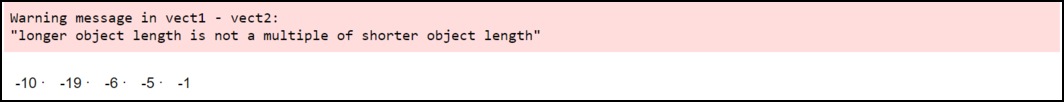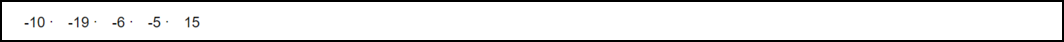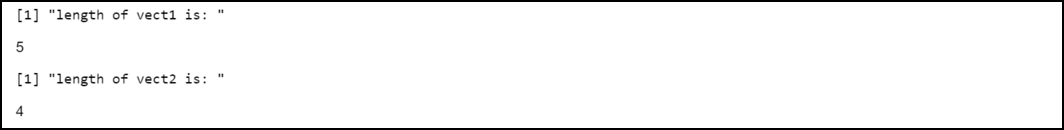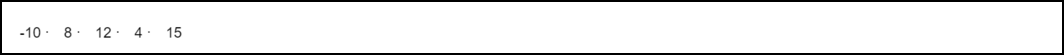GFG App
Open AppBrowser
Continue

# How to Fix in R: longer object length is not a multiple of shorter object length

In this article, we will be looking at the approach to fix the “longer object length is not a multiple of shorter object length” error in the R programming language.

This is a warning message produced by the R compiler. The complete form of this warning message is given below:

```Warning message:
In vect1 + vect2 :
longer object length is not a multiple of shorter object length```

Reason: Such an error might occur in R when we try to do some operations on the vectors having unequal lengths.

## When this error may occur:

Consider that we have two vectors vect1 and vect2. Both contain 5 and 4 elements respectively.

## R

 `# Define two vectors ` `vect1 <- ``c``(6, 8, 12, 4, 15) ` `vect2 <- ``c``(16, 27, 18, 9)`

Example:

Now let’s try to subtract and print corresponding values.

## R

 `# Define two vectors ` `vect1 <- ``c``(6, 8, 12, 4, 15) ` `vect2 <- ``c``(16, 27, 18, 9) ` ` `  `# Subtract and display result ` `vect1 - vect2`

Output:Error

Interpretation of output:

The output shows the difference of the corresponding values in each vector. We received the warning message because the 2 vectors are of unequal length. Note that the R compiler uses the difference between the last element of vect1 and also the first element of vect2. Hence, we will say that the values are repeated in a very circular form.

When we are unaware of the particular lengths of the vectors. Then, it’s advisable to test the lengths of the vectors beforehand. In R we’ve got the length() function that’s accustomed to determine the number of elements present in vectors.

## How to fix this error:

We have to make sure that the length of vectors with which we are going to deal with must have the same length. For example, in the above source code vector2 is one length shorter than vect1. Hence we can insert 0 at the end of vect2.

Example:

## R

 `# Define two vectors ` `vect1 <- ``c``(6, 8, 12, 4, 15) ` `vect2 <- ``c``(16, 27, 18, 9) ` ` `  `# Insert a zero ` `vect2 <- ``c``(vect2, 0) ` ` `  `# Subtract and display result ` `vect1 - vect2`

Output:Output

As you can see in the output this time the program compiled successfully without throwing any warning message.

Example:

Here, we are simply printing the length of both the vector provided using the length() function in R.

## R

 `# Define two vectors ` `vect1 <- ``c``(6, 8, 12, 4, 15) ` `vect2 <- ``c``(16, 27, 18, 9) ` ` `  `# Print the length of vect1 ` `print``(``"length of vect1 is: "``) ` `length``(vect1) ` ` `  `# Print the length of vect2 ` `print``(``"length of vect2 is: "``) ` `length``(vect2)`

Output:Output

Example:

If the difference in the length of the vectors is quite large then we can use a for-loop and add those many 0’s at the end of the shorter vector.

## R

 `# Define two vectors ` `vect1 <- ``c``(6, 8, 12, 4, 15) ` `vect2 <- ``c``(16) ` ` `  `# Insert zeros to the end of vector vect2 ` `for``(i ``in ``((``length``(vect2)+1):``length``(vect1))) ` `  ``+{vect2 = ``c``(vect2, 0)} ` ` `  `# Subtract and display result ` `vect1 - vect2`

Output:Output

My Personal Notes arrow_drop_up